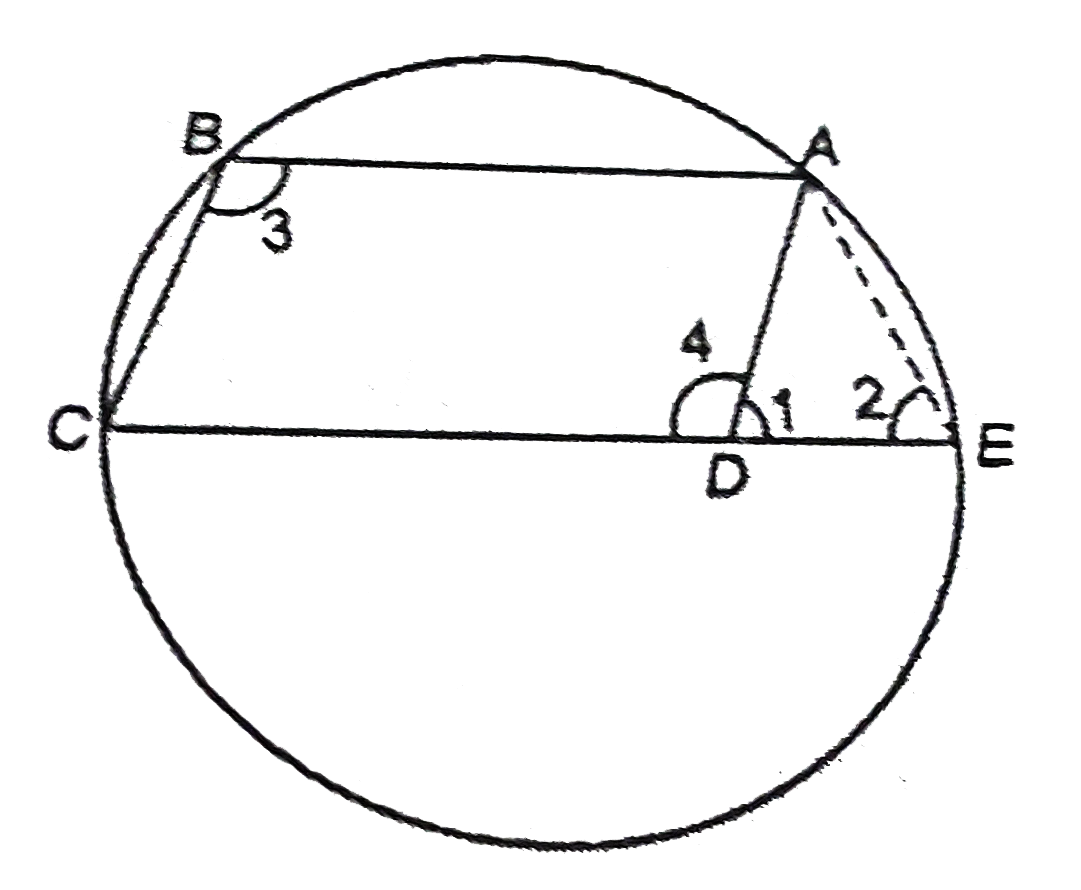# ABCD is a parallelogram . The circle passing through the vertices. A, B and C intersects CD (or CD produced) at E. Prove that AE=AD.

40 views

closed
ABCD is a parallelogram . The circle passing through the vertices. A, B and C intersects CD (or CD produced) at E. Prove that AE=AD.

by (88.7k points)
selected by

angle1 +angle4 =180^@ (L.P.A.) ......(1)
angle 2+angle3 =180^@ ........(2)
(sum of opposite of a cyclic quadrilateral is 180^@)
therefore From (1) and (2), we get
angle1 +angle4 =angle2 +anlge3 ......(3)
But (opposite angles of a parallelogram )
therefore From (3) and (4) ,
angle1 +angle4 =angle2 +angle4
rArrangle1 =angle2
rArrAE=AD" "(because" sides opposite to equal angles are equal")